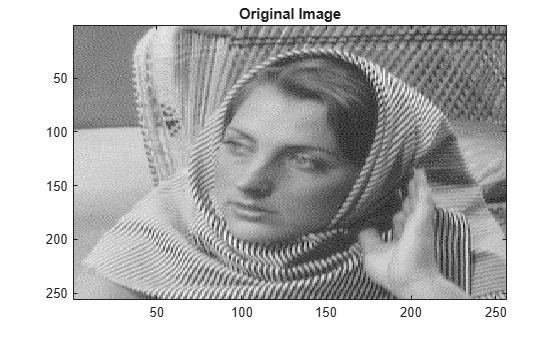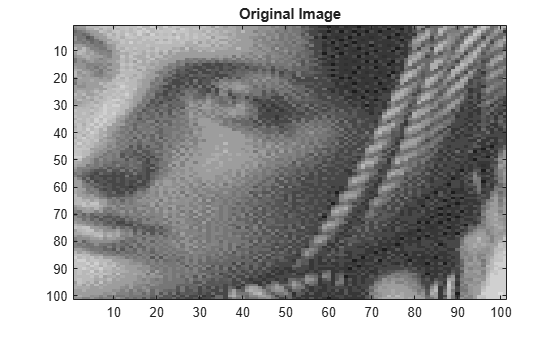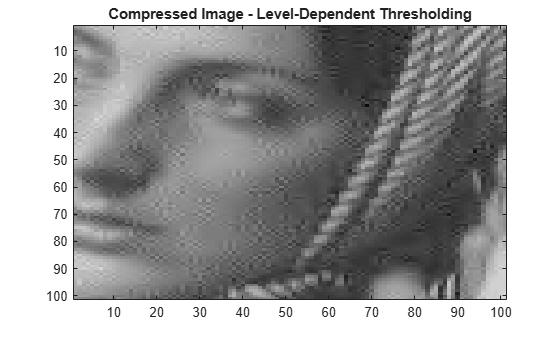Documentation

### This is machine translation

Mouseover text to see original. Click the button below to return to the English version of the page.

To view all translated materials including this page, select Country from the country navigator on the bottom of this page.

## Data Compression using 2D Wavelet Analysis

The purpose of this example is to show how to compress an image using two-dimensional wavelet analysis. Compression is one of the most important applications of wavelets. Like de-noising, the compression procedure contains three steps:

• Decompose: Choose a wavelet, choose a level N. Compute the wavelet decomposition of the signal at level N.

• Threshold detail coefficients: For each level from 1 to N, a threshold is selected and hard thresholding is applied to the detail coefficients.

• Reconstruct: Compute wavelet reconstruction using the original approximation coefficients of level N and the modified detail coefficients of levels from 1 to N.

The difference with the de-noising procedure is found in step 2. There are two compression approaches available:

• The first consists of taking the wavelet expansion of the signal and keeping the largest absolute value coefficients. In this case, you can set a global threshold, a compression performance, or a relative square norm recovery performance. Thus, only a single parameter needs to be selected.

• The second approach consists of applying visually determined level-dependent thresholds.

### Load an Image

Let us examine a real-life example of compression for a given and unoptimized wavelet choice, to produce a nearly complete square norm recovery for an image.

```load woman; % Load original image. image(X) title('Original Image') colormap(map)````x = X(100:200,100:200); % Select ROI`

### Method 1: Global Thresholding

The compression features of a given wavelet basis are primarily linked to the relative scarceness of the wavelet domain representation for the signal. The notion behind compression is based on the concept that the regular signal component can be accurately approximated using the following elements: a small number of approximation coefficients (at a suitably chosen level) and some of the detail coefficients.

```n = 5; % Decomposition Level w = 'sym8'; % Near symmetric wavelet [c l] = wavedec2(x,n,w); % Multilevel 2-D wavelet decomposition.```

In this first method, the WDENCMP function performs a compression process from the wavelet decomposition structure [c,l] of the image.

```opt = 'gbl'; % Global threshold thr = 20; % Threshold sorh = 'h'; % Hard thresholding keepapp = 1; % Approximation coefficients cannot be thresholded [xd,cxd,lxd,perf0,perfl2] = wdencmp(opt,c,l,w,n,thr,sorh,keepapp); image(x) title('Original Image') colormap(map)``````figure('Color','white'),image(xd) title('Compressed Image - Global Threshold = 20') colormap(map)```L2-norm recovery (%)

`perf0`
```perf0 = 74.3067 ```

Compression score (%)

`perfl2`
```perfl2 = 99.9772 ```

The density of the current decomposition sparse matrix is:

```cxd = sparse(cxd); cxd_density = nnz(cxd)/prod(size(cxd))```
```cxd_density = 0.2569 ```

### Method 2: Level-Dependent Thresholding

The WDENCMP function also allows level and orientation-dependent thresholds. In this case the approximation is kept. The level-dependent thresholds in the three orientations horizontal, diagonal and vertical are as follows:

```opt = 'lvd'; % Level dependent thresholds thr_h = [17 18]; % Horizontal thresholds. thr_d = [19 20]; % Diagonal thresholds. thr_v = [21 22]; % Vertical thresholds. thr = [thr_h ; thr_d ; thr_v];```

In this second example, notice that the WDENCMP function performs a compression process from the image x.

```[xd2,cxd2,lxd2,perf02,perfl22] = wdencmp(opt,x,w,2,thr,sorh); image(x) title('Original Image') colormap(map)``````figure('Color','white'),image(xd2) title('Compressed Image - Level-Dependent Thresholding') colormap(map)```L2-norm recovery (%)

`perf02`
```perf02 = 77.3435 ```

Compression score (%)

`perfl22`
```perfl22 = 99.6132 ```

The density of the current decomposition sparse matrix is:

```cxd2 = sparse(cxd2); cxd2_density = nnz(cxd2)/prod(size(cxd2))```
```cxd2_density = 0.2266 ```

### Summary

By using level-dependent thresholding, the density of the wavelet decomposition was reduced by 3% while improving the L2-norm recovery by 3%. If the wavelet representation is too dense, similar strategies can be used in the wavelet packet framework to obtain a sparser representation. You can then determine the best decomposition with respect to a suitably selected entropy-like criterion, which corresponds to the selected purpose (de-noising or compression).

##### SupportGet trial now